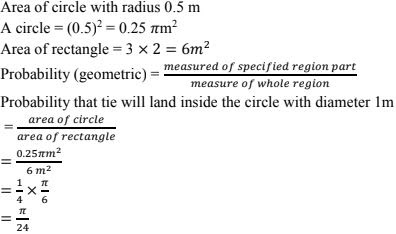# Chapter 13 Probability RD Sharma Solutions Exercise 13.2 Class 10 Maths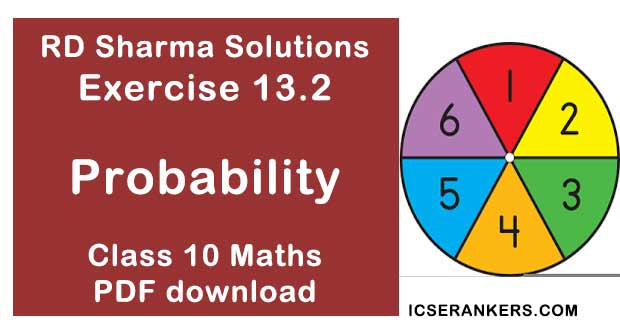Chapter Name RD Sharma Chapter 13 Probability Book Name RD Sharma Mathematics for Class 10 Other Exercises Exercise 13.1 Related Study NCERT Solutions for Class 10 Maths

### Exercise 13.2 Solutions

1. In the accompanying diagram a fair spinner is placed at the center O of the circle. Diameter AOB and radius OC divide the circle into three regions labelled X, Y and Z. If ∠BOC = 45°. What is the probability that the spinner will land in the region X? (See fig)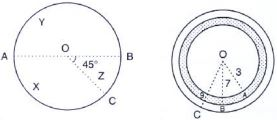Solution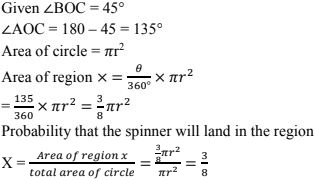2. A target shown in Fig. below consists of three concentric circles of radii, 3, 7 and 9 cm respectively. A dart is thrown and lands on the target. What is the probability that the dart will land on the shaded region?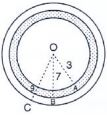Solution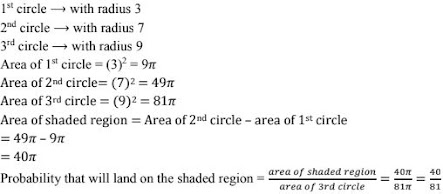3. In below Fig., points A, B, C and D are the centers of four circles that each have a radius of length one unit. If a point is selected at random from the interior of square ABCD. What is the probability that the point will be chosen from the shaded region?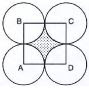Solution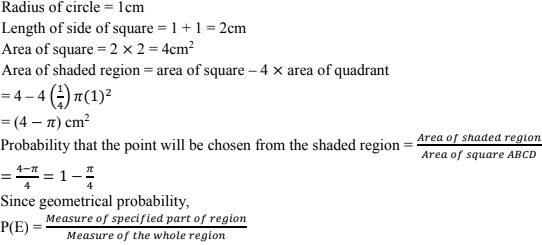4. In the Fig. below, JKLM is a square with sides of length 6 units. Points A and B are the mid- points of sides KL and LM respectively. If a point is selected at random from the interior of the square. What is the probability that the point will be chosen from the interior of ∆JAB?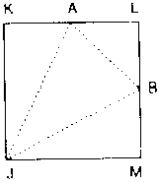Solution
Length of side of square JKLM = 6 cm
Area of square JKLM = 62 = 36 cm2
Since A & B are the mid points of KL & LM
KA = AL = LB = LM = 3 cm
Area of ∆ AJB = area of square – area of ∆ AKJ – area of ∆ ALB – area of ∆ BMJ
= 36 − 1/2 ×6 ×3 - 1/2 ×6 ×3
= 36 − 9 − 4.5 − 9
= 13.5 sq. units
Probability that the point will be chosen from the interior of ∆AJB = Area of ∆AJB/Area of square

5. In the Fig. below, 13, a square dart board is shown. The length of a side of the larger square
is 1.5 times the length of a side of the smaller square. If a dart is thrown and lands on the
larger square. What is the probability that it will land in the interior of the smaller square?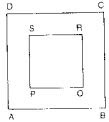Solution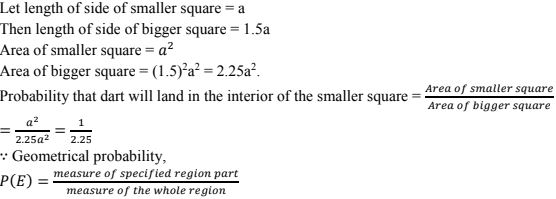6. Suppose you drop a tie at random on the rectangular region shown in Fig. below. What is
the probability that it will land inside the circle with diameter 1 m?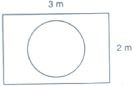Solution